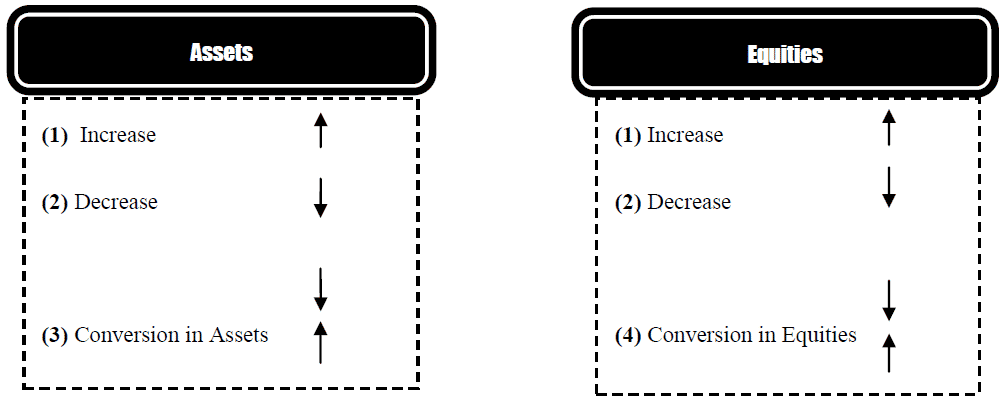Select Page

# Accounting Equation

Previous Lesson: Types of Accounts

Next Lesson: Accounting Variation Proforma

Accounting equation is a basic concept of agreement between left-hand and right-hand site and starting pint of double entry. Double entry bookkeeping states that for every debit entry there should be pass a credit entry. Every transaction has twofold effect; this concept has a result of Balance Sheet Equation or Fundamental Equation. At any point of time total assets must be equal to equities. In other words we can say that left hand side which is resource side must be equal to right hand side which is of course source side.

The two basic elements of a business are what it owns and what it owes. Assets are the resources a business owns. For example, ABC Ltd. has total assets of approximately Rs. 50.5 billion. Liabilities and owner’s equity are the rights or claims against these resources. Thus, ABC Ltd. has Rs. 50.5 billion of claims against its Rs. 50.5 billion of assets. Claims of those to whom the company owes money (creditors) are called liabilities. Claims of owners are called owner’s equity. ABC Ltd. has liabilities of Rs. 20 billion and owners’ equity of Rs. 30.5 billion. We can express the relationship of assets, liabilities, and owner’s equity as an equation, as shown:

#### Left hand side = Right hand sideThis relationship is the basic accounting equation. Assets must equal the sum of liabilities and owner’s equity. Liabilities appear before owner’s equity in the basic accounting equation because they are paid first if a business is liquidated. The accounting equation applies to all economic entities regardless of size, nature of business, or form of business organization. The equation provides the underlying framework for recording and summarizing economic events.

## Different Presentation of Accounting Equation

There are different style of presentation mention below for balance sheet equation:The accounting equation as a statement of financial position may be expressed as:

Assets minus Liabilities equal Ownership interest; the ownership interest is the residual claim after liabilities to third parties have been satisfied. The equation expressed in this form emphasizes that residual aspect. Another way of thinking about an equation is to imagine a balance with a bucket on each end. In one bucket are the assets (A) minus liabilities (L). In the other is the ownership interest (OI). If anything happens to disturb the assets then the balance will tip unevenly unless some matching disturbance is applied to the ownership interest. If anything happens to disturb the liabilities then the balance will tip unevenly unless some matching disturbance is applied to the ownership interest. If a disturbance applied to an asset is applied equally to a liability, then the balance will remain level.

## Four Effects of Transactions

Accounting Transaction is broadly classified into four categories:

####>>> Practice Questions with the help of Accounting Equation Format.

## Accounting Equation Examples

### Example # 1:

For each of the following transactions indicate the effects i.e. (Increase, Decrease, Conversion or No Effect)?>>> Try more Accounting Equation Problems and Solutions.

### Example # 2:

From the list of accounts below, determine which assets are and which equities are.  List the assets under the Asset Column and the equities under the equities Column.  Then add each column and complete the Fundamental Accounting Equation?

 Accounts Asset Equities Cash Rs. 5,000 Rs. 5,000 Accounts Receivable 10, 600 10,600 Building 35,000 35,000 Accounts Payable 5,000 5,000 Inventory 200 200 Computer Equipment 18,000 18,000 Bank Loan 6,000 6,000 Mortgage Payable 10,000 10,000 Owner Equity 47,800 47,800 TOTAL Rs. 137,600 Rs. 68,800 Rs. 68,800

>>> Try more Accounting Equation Examples

### Example # 3:

For each of the following equations, a figure is missing.  In the space provided, please supply the missing figure that will balance the equation.

 Assets Liabilities Owner’s Equity 100 20.00 80 790 720 70 2,000 400 1,600 11,218 10,000 1,218 1,010 25 985 340 12 328 8793 0 8793 2,000 1,501 499

>> Practice Accounting Equation Quiz 1 and Quiz 2.

## Expanded Accounting Equation

The Expanded Accounting Equation of sole proprietorship and partnership is Accounting Equation (Assets = Liabilities + Owner’s Equity) – Expenses + Revenue – Drawings Account.

In case of company business the expanded accounting equation in the balance sheet equation (Assets = Liabilities + Stockholders’ Equity) with replacement of Stockholders’ Equity with Paid-in Capital – Expenses + Revenues – Treasury Stock – Dividends.

> > Practice Accounting Equation Problems and Solutions.

Previous Lesson: Types of Accounts

Next Lesson: Accounting Variation Proforma

## References

Mukharji, A., & Hanif, M. (2003). Financial Accounting (Vol. 1). New Delhi: Tata McGraw-Hill Publishing Co.

Narayanswami, R. (2008). Financial Accounting: A Managerial Perspective. (3rd, Ed.) New Delhi: Prentice Hall of India.

Ramchandran, N., & Kakani, R. K. (2007). Financial Accounting for Management. (2nd, Ed.) New Delhi: Tata McGraw Hill.

1.great indeed , this is very helpful THUMBS UP if you find this useful through your studying.

2.This is very interesting, You’re a very skilled blogger. I have joined your rss feed and look forward to seeking more of your magnificent post. Also, I have shared your website in my social networks!

3.Somebody necessarily help to make seriously posts I’d state. This is the first time I frequented your web page and to this point? I amazed with the research you made to create this particular post extraordinary. Wonderful job!

4.I think this is a real great article.Thanks Again.

5.Your style is so unique in comparison to other people I have read stuff from. Thanks for posting when you have the opportunity, Guess I will just bookmark this blog.

6.Accounting equation is important topic for beginner of accounting students. This page give me valuable insights. Thank you so much.

7.8.